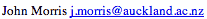Data Structures and Algorithms Matrix Chain Multiplication

#### Problem

We are given a sequence of matrices to multiply:
A1 A2 A3 ... An
Matrix multiplication is associative, so
A1 ( A2 A3 ) = ( A1 A2 ) A3
that is, we can can generate the product in two ways.

The cost of multiplying an nxm by an mxp one is O(nmp) (or O(n3) for two nxn ones). A poor choice of parenthesisation can be expensive: eg if we have

MatrixRowsColumns
A110100
A21005
A3550
the cost for ( A1 A2 ) A3 is
 A1A2 10x100x5 = 5000 => A1 A2 (10x5) (A1A2) A3 10x5x50 = 2500 => A1A2A3 (10x50) Total 7500
but for A1 ( A2 A3 )
 A2A3 100x5x50 = 25000 => A2A3 (100x50) A1(A2A3) 10x100x50 = 50000 => A1A2A3 (10x50) Total 75000
Clearly demonstrating the benefit of calculating the optimum order before commencing the product calculation!

### Optimal Sub-structure

As with the optimal binary search tree, we can observe that if we divide a chain of matrices to be multiplied into two optimal sub-chains:
(A1 A2 A3 ... Aj) (Aj+1 ... An )
then the optimal parenthesisations of the sub-chains must be composed of optimal chains. If they were not, then we could replace them with cheaper parenthesisations.

This property, known as optimal sub-structure is a hallmark of dynamic algorithms: it enables us to solve the small problems (the sub-structure) and use those solutions to generate solutions to larger problems.

For matrix chain multiplication, the procedure is now almost identical to that used for constructing an optimal binary search tree. We gradually fill in two matrices,

• one containing the costs of multiplying all the sub-chains. The diagonal below the main diagonal contains the costs of all pair-wise multiplications: cost[1,2] contains the cost of generating product A1A2, etc. The diagonal below that contains the costs of triple products: eg cost[1,3] contains the cost of generating product A1A2A3, which we derived from comparing cost[1,2] and cost[2,3], etc.
• one containing the index of last array in the left parenthesisation (similar to the root of the optimal sub-tree in the optimal binary search tree, but there's no root here - the chain is divided into left and right sub-products), so that best[1,3] might contain 2 to indicate that the left sub-chain contains A1A2 and the right one is A3 in the optimal parenthesisation of A1A2A3.
As before, if we have n matrices to multiply, it will take O(n) time to generate each of the O(n2) costs and entries in the best matrix for an overall complexity of O(n3) time at a cost of O(n2) space.

### Animation

Matrix Chain Multiplication Animation
This animation was written by Woi Ang.
If you don't have a high resolution display, the bottom of the screen will be clipped!©, 1998